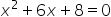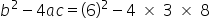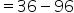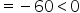Mathematics
Easy

Question

# How many solutions are there for the equation 3using the discriminant?

## 0    1    2    Either 1 or 2Hint:

## The correct answer is: 0

### Step 1 of 1:We have given a quadratic  equation 3The discriminant is less than zero, so it has no real solutions.

The discriminant is the part of the quadratic formula found within the square root.

### Related Questions to study#### With Turito Foundation.#### Get an Expert Advice From Turito.